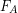# 6.8: Unit 6 Practice and Assessment

$$\newcommand{\vecs}{\overset { \rightharpoonup} {\mathbf{#1}} }$$ $$\newcommand{\vecd}{\overset{-\!-\!\rightharpoonup}{\vphantom{a}\smash {#1}}}$$$$\newcommand{\id}{\mathrm{id}}$$ $$\newcommand{\Span}{\mathrm{span}}$$ $$\newcommand{\kernel}{\mathrm{null}\,}$$ $$\newcommand{\range}{\mathrm{range}\,}$$ $$\newcommand{\RealPart}{\mathrm{Re}}$$ $$\newcommand{\ImaginaryPart}{\mathrm{Im}}$$ $$\newcommand{\Argument}{\mathrm{Arg}}$$ $$\newcommand{\norm}{\| #1 \|}$$ $$\newcommand{\inner}{\langle #1, #2 \rangle}$$ $$\newcommand{\Span}{\mathrm{span}}$$ $$\newcommand{\id}{\mathrm{id}}$$ $$\newcommand{\Span}{\mathrm{span}}$$ $$\newcommand{\kernel}{\mathrm{null}\,}$$ $$\newcommand{\range}{\mathrm{range}\,}$$ $$\newcommand{\RealPart}{\mathrm{Re}}$$ $$\newcommand{\ImaginaryPart}{\mathrm{Im}}$$ $$\newcommand{\Argument}{\mathrm{Arg}}$$ $$\newcommand{\norm}{\| #1 \|}$$ $$\newcommand{\inner}{\langle #1, #2 \rangle}$$ $$\newcommand{\Span}{\mathrm{span}}$$$$\newcommand{\AA}{\unicode[.8,0]{x212B}}$$

### Outcome 1

1) For each object below, draw a free body diagram:

• A car hanging from a crane (there are two forces).
• A car skidding to a stop (there are three forces).
• A car with the parking brake set being pushed on by a someone, but not moving (there are four forces here, but two of them are the same type).

2) A person stands on a scale. What type of force is pulling them down? What type of force is provided by the scale to hold them up?

### Outcome 2

4) Consider the following items:

• Pliers
• Tweezers
• Shovel

(a) For each case, draw a stick figure of the tool and label the fulcrum, effort, load, effort arm, and load arm.

(b) State the class of lever for each item above.

(c) For each item above, state whether the tool is providing mechanical advantage or increasing range of motion.

### Outcome 3

5) When a person raises their heels off the ground, the foot acts like a lever.

(a) Typically we consider the foot as a second class lever, but if we treat the ankle bone as the fulcrum, the tension in the calf muscle as the effort, and the normal force from the floor as the resistance, what class of lever is this system?

(b) Calculate the tension applied by the calf muscles () to lift a person with weight of 637 N.

(c) Calculate the force in the ankle joint between the foot and the lower leg bones (). [Hint: Both the normal force from the floor and the calf tension point upward. In order for the foot to be in static equilibrium, the force of the lower leg pushing down on the foot must cancel out both of those upward forces.]The foot acting as a lever arm. Image Credit: OpenStax College Physics

6) The head and neck are also a lever system.

(a) State the class of this lever system.

(b) Calculate the force of tension in the neck muscles () to hold the head in the position shown in the diagram.

(c) Calculate the force on the head-neck joint ().The head and neck acting as a lever system.Image Credit: OpenStax College Physics

## Outcome 4

7) Find a value for the kinetic coefficient of friction between ends of a bone in a synovial joint lubricated by synovial fluid. State your source.

8) If the normal force between bones in the knee is 160 lbs, what is the kinetic frictional force between the surfaces of the knee bones?

9) A car with 10,000 N weight is sitting on concrete with the parking brake on,

a) What is the normal force on the car from the concrete? [Hint: Is the car in static equilibrium?]

b) What is the maximum horizontal force that can be applied before the car begins to skid? List your sources for the friction coefficient.

c) After the car begins to skid, how much force is required to keep it moving at constant speed, despite friction?

d) If you apply only 120 N of horizontal force to the stationary car, what is the static frictional force at that time?

1. OpenStax, College Physics. OpenStax CNX. Aug 3, 2018 http://cnx.org/contents/031da8d3-b525-429c-80cf-6c8ed997733a@11.42
2. OpenStax, College Physics. OpenStax CNX. Aug 3, 2018 http://cnx.org/contents/031da8d3-b525-429c-80cf-6c8ed997733a@11.42

This page titled 6.8: Unit 6 Practice and Assessment is shared under a CC BY-NC-SA 4.0 license and was authored, remixed, and/or curated by Lawrence Davis (OpenOregon) via source content that was edited to the style and standards of the LibreTexts platform; a detailed edit history is available upon request.